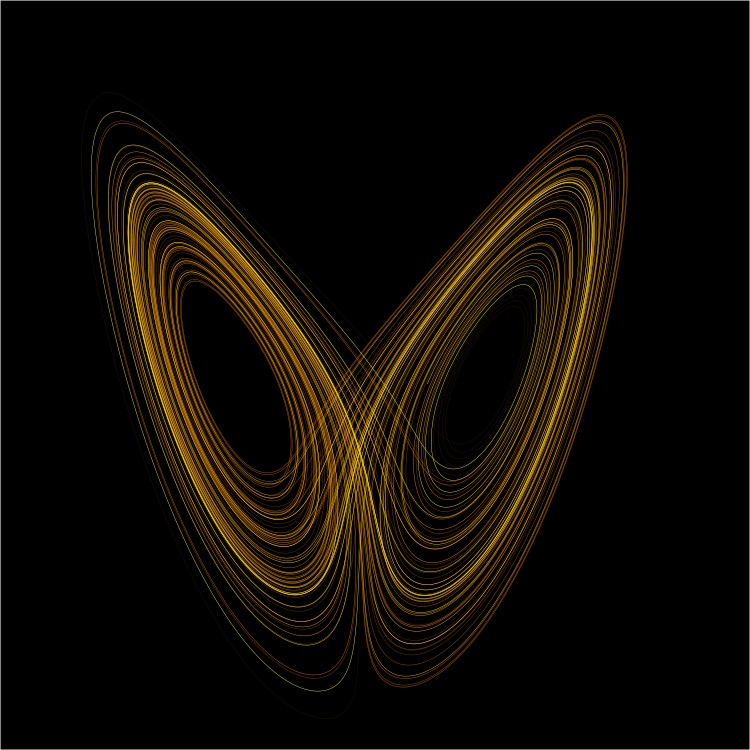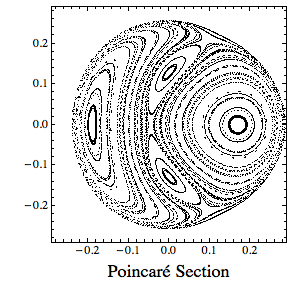# Order and Chaos

In Computation by Brian Koberlein0 Comments

A recurring theme in computational astrophysics (and physics in general) is the concept of chaos.  While many aspects of the universe are ordered and predictable, other aspects are quite chaotic.  Often things lie at a fine line between the two.

A good example of this can be seen in galactic motion.  On the one hand things are quite regular.  At a broad level stars move in a generally circular path around the galactic center.  This is analogous to our solar system, where planets move in (roughly) circular orbits around the sun.  This makes it easy to make a rough model of our galaxy as a fairly uniform disk of stars.

Of course when we look more closely things are not so simple.  For one our galaxy is not a uniform disk of stars, but rather lies mainly in spiral arms.  (Why this is the case is a topic for a future post.)  Then there is the motion of individual stars and star clusters themselves.

It turns out the motion of stars can be approximately described by a simple differential equation called the Henon-Heiles equation.  Unfortunately the solution to this equation is chaotic.  In other words the solution is very dependent on a star’s initial velocity and position.  Determining precise measurements of a star’s position and velocity can be quite a challenge.  So usually we have to look at general properties of the solution rather than finding a particular solution.The good news is that the Henon-Heiles equation as long been studied by mathematicians, so we actually know a great deal about it.  One common way to look at general solutions is to plot what is known as a Poincare map of solutions.  I’ve plotted one for the Henon-Heiles here.  A Poincare map help you determine certain aspects of a star’s motion.  For example, in the figure below you can see that the range is bounded to a particular region.  So we know the star won’t just wander off.  We can also see regions where the motion tends to cluster.  So even though we don’t know the exact motion of a star, we know its general motion.

Problems like this can’t be solved well analytically, so it is an area where computational methods shine.